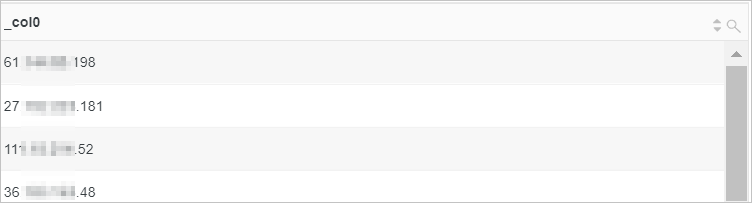This topic describes the syntax and examples of conditional expressions.

## CASE WHEN syntax

The CASE WHEN syntax is used to classify continuous data.

• Syntax
``````CASE WHEN condition1 THEN result1
[WHEN condition2 THEN result2]
[ELSE result3]
END``````
• Example
• Extract browser information from the value of the http_user_agent field, classify the information into Chrome, Safari, and unknown types, and calculate the number of PVs for the three types.
• Query statement
``````* | SELECT CASE
WHEN http_user_agent like '%Chrome%' then 'Chrome'
WHEN http_user_agent like '%Safari%' then 'Safari'
ELSE 'unknown'
END AS http_user_agent,
count(*) AS pv
GROUP BY http_user_agent``````
• Query and analysis results• Query the distribution of different request times.
• Query statement
``````* | SELECT
CASE
WHEN request_time < 10 then 't10'
WHEN request_time < 100 then 't100'
WHEN request_time < 1000 then 't1000'
WHEN request_time < 10000 then 't10000'
ELSE 'large' END
AS request_time,
count(*) AS pv
GROUP BY request_time``````
• Query and analysis results## Syntax for the if() function

Syntax for the if() function is used to categorize data, similar to CASE WHEN syntax.

• Syntax
• If the condition is true, the true_value column is returned. Otherwise, null is returned.
``if(condition, true_value)``
• If the condition is true, the true_value column is returned. Otherwise, the false_value column is returned.
``if(condition, true_value, false_value)``
• Example

Calculate the ratio of requests whose status code is 200 to all requests

• Query statement
``* | SELECT sum(if(status =200,1,0))*1.0 / count(*) AS status_200_percentage``
• Query and analysis results## COALESCE syntax

The COALESCE syntax is used to return the first non-null value in multiple columns.
• Syntax
``coalesce(expression1, expression2, expression3, expression4)``
• Example

Calculate the ratio of the expenses of yesterday to the expenses of the same day last month.

• Query statement
``* | SELECT compare("expenses of Yesterday", 604800) AS diff FROM (SELECT Coalesce (sum(PretaxAmount), 0) AS "expenses of Yesterday" FROM website_log)``
• Query and analysis results• The value 6514393413.0 indicates the expenses of yesterday.
• The value 19578267596.0 indicates the expenses of the same day last month.
• The value 0.33273594719539659 indicates the ratio of the expenses of yesterday to the expenses of the same day last month.

## NULLIF syntax

The NULLIF syntax is used to check whether the values of two columns are the same. If the values are the same, null is returned. Otherwise, the value of expression1 is returned.

• Syntax
``nullif(expression1, expression2)``
• Example
Check whether the values of the client_ip and host fields are the same.
• Query statement
``* | SELECT nullif(client_ip,host)``
• Query and analysis results

If the values of the client_ip, host fields are different, the value of the client_ip field is returned.## TRY syntax

The TRY syntax is used to capture errors to ensure that the system can continue to query and analyze data.

• Syntax
``try(expression)``
• Example
If an error occurs when the regexp_extract function is invoked, the try() function captures the error and continue to query and analyze data. The query and analysis results are returned.
• Query statement
``* | SELECT try(regexp_extract(request_uri, '.*\/(file. *)', 1)) AS file, count(*) AS count GROUP BY file``
• Query and analysis results